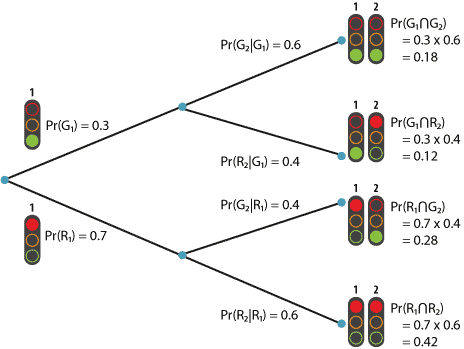## Content

### Tree diagrams

Probability problems sometimes involve more than one step or stage, and at the different stages various outcomes may occur. It can be tricky to keep track of the stages, outcomes and probabilities, and a tree diagram' is often helpful. Such a diagram is usually labelled in a sensible way with the outcomes that may occur at each stage and their probabilities. The probabilities of the outcomes at the ends of the branches of the tree may be found using the multiplication rule.

#### Example: Traffic lights

Rose rides a bicycle to work. There is a sequence of two sets of traffic lights on her route, not far apart. For simplicity, we ignore amber and assume the lights are only red or green. Suppose the probability that the first set is green when she arrives at it is 0.3. If she gets through on green at the first set, the probability that the second set is green when she arrives is 0.6; if she has to stop on red at the first set, the probability that the second set is green when she arrives is 0.4. What is the probability that she gets through both sets on green?

Define $$G_i$$ to be the event that set $$i$$ is green on her arrival, and similarly for $$R_i$$ (red at set $$i$$). We need to find $$\Pr(G_1 \cap G_2)$$; note how the word both' in the question translates to a calculation of the probability of an intersection between two events. We use the multiplication theorem:

$\Pr(G_1 \cap G_2) = \Pr(G_1) \Pr(G_2|G_1) = 0.3 \times 0.6 = 0.18.$

In this calculation it is important to recognise the conditional probabilities we are given. `If she gets through on green at the first set' is language that tells us that a conditional probability is being provided, the condition being the event $$G_1$$, that is, she meets a green light at the first set.

There are several things that can happen, as shown in the following tree diagram.Tree diagram for the traffic-lights example.
Detailed description

With the probabilities at the first stage and the conditional probabilities at the second stage clearly labelled, it is a straightforward exercise to move along the branches of the tree, multiplying, to arrive at the probabilities of outcomes at the end of the branches; each case uses the multiplication theorem. The outcomes at the ends of the branches are intersections between events at the first and second stages.

What is the probability that the second set is green when she meets it? Note that the problem, as posed, does not specify this. We are only given conditional probabilities for the second set of lights. However, we can obtain the probability $$\Pr(G_2)$$ that she meets green at the second set by considering the two events $$G_1 \cap G_2$$ and $$R_1 \cap G_2$$. These are mutually exclusive, and $$G_2 = (G_1 \cap G_2) \cup (R_1 \cap G_2)$$. Hence $$\Pr(G_2) = 0.18 + 0.28 = 0.46$$.

Note that the events $$G_1$$ and $$G_2$$ are not independent, since

$\Pr(G_1 \cap G_2) = 0.18 \qquad \text{and} \qquad \Pr(G_1) \Pr(G_2) = 0.3 \times 0.46 = 0.138,$ and therefore $$\Pr(G_1 \cap G_2) \neq \Pr(G_1) \Pr(G_2)$$.

Exercise 12

A player pays $4 to play the following game. A fair die is rolled once. If the outcome is not a prime number, the player loses. If a prime number is rolled (2, 3 or 5), the die is rolled again. If the outcome of the second roll is not a prime number, the player loses. If a prime number is rolled the second time, the player is paid an amount (in dollars) equal to the sum of the outcomes of the two rolls of the die. Draw the applicable tree diagram and answer the following: 1. What is the probability of losing at the first roll? 2. What is the probability of winning$6?
3. What is the probability of winning?

Next page - Content - The law of total probability